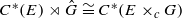Hostname: page-component-546b4f848f-gfk6d Total loading time: 0 Render date: 2023-06-04T21:50:47.838Z Has data issue: false Feature Flags: { "useRatesEcommerce": true } hasContentIssue false

# Group actions on topological graphs

Published online by Cambridge University Press:  16 September 2011

## Abstract

We define the action of a locally compact group G on a topological graph E. This action induces a natural action of G on the C*-correspondence ℋ(E) and on the graph C*-algebra C*(E). If the action is free and proper, we prove that C*(E)⋊rG is strongly Morita equivalent to C*(E/G) . We define the skew product of a locally compact group G by a topological graph E via a cocycle c:E1G. The group acts freely and properly on this new topological graph E×cG. If G is abelian, there is a dual action on C* (E) such that. We also define the fundamental group and the universal covering of a topological graph.

Type
Research Article
Information
Ergodic Theory and Dynamical Systems , October 2012 , pp. 1527 - 1566

## Access options

Get access to the full version of this content by using one of the access options below. (Log in options will check for institutional or personal access. Content may require purchase if you do not have access.)

## References

[ADR00]Anantharaman-Delaroche, C. and Renault, J.. Amenable Groupoids (Monographs of L’Enseignement Mathématique, 36). L’Enseignement Mathématique, Geneva, 2000, with a foreword by Georges Skandalis and Appendix B by E. Germain.Google Scholar
[Bau93]Baumslag, G.. Topics in Combinatorial Group Theory (Lectures in Mathematics ETH Zürich). Birkhäuser, Basel, 1993.CrossRefGoogle Scholar
[BH99]Bridson, M. R. and Haefliger, A.. Metric spaces of non-positive curvature (Grundlehren der Mathematischen Wissenschaften, 319). Springer, Berlin, 1999.CrossRefGoogle Scholar
[BO08]Brown, N. P. and Ozawa, N.. C *-algebras and finite-dimensional approximations (Graduate Studies in Mathematics, 88). American Mathematical Society, Providence, RI, 2008.CrossRefGoogle Scholar
[EKQR06]Echterhoff, S., Kaliszewski, S., Quigg, J. and Raeburn, I.. A Categorical Approach to Imprimitivity Theorems for C *-Dynamical Systems, Vol. 180 (Memoires of the American Mathematical Society, 850). American Mathematical Society, Providence, RI, 2006.Google Scholar
[FLR00]Fowler, N. J., Laca, M. and Raeburn, I.. The C *-algebras of infinite graphs. Proc. Amer. Math. Soc. 128 (2000), 23192327.CrossRefGoogle Scholar
[GT01]Gross, J. L. and Tucker, T. W.. Topological Graph Theory. Dover Publications Inc., Mineola, NY, 2001, reprint of the 1987 original [Wiley, New York] with a new preface and supplementary bibliography.Google Scholar
[HN08]Hao, G. and Ng, C.-K.. Crossed products of C *-correspondences by amenable group actions. J. Math. Anal. Appl. 345(2) (2008), 702707.CrossRefGoogle Scholar
[HRW05]an Huef, A., Raeburn, I. and Williams, D. P.. A symmetric imprimitivity theorem for commuting proper actions. Canad. J. Math. 57 (2005), 9831011.CrossRefGoogle Scholar
[Hus94]Husemoller, D.. Fibre Bundles, 3rd edn(Graduate Texts in Mathematics, 20). Springer, New York, 1994.CrossRefGoogle Scholar
[KQR97]Kaliszewski, S., Quigg, J. and Raeburn, I.. Duality of restriction and induction for C *-coactions. Trans. Amer. Math. Soc. 349 (1997), 20852113.CrossRefGoogle Scholar
[KQR08]Kaliszewski, S., Quigg, J. and Raeburn, I.. Proper actions, fixed-point algebras and naturality in nonabelian duality. J. Funct. Anal. 254 (2008), 29492968.CrossRefGoogle Scholar
[KQR11]Kaliszewski, S., Quigg, J. and Raeburn, I.. Skew products and coactions for topological graphs. Preprint, 2011.Google Scholar
[Kas88]Kasparov, G. G.. Equivariant KK-theory and the Novikov conjecture. Invent. Math. 91(1) (1988), 147201.CrossRefGoogle Scholar
[Kat02]Katsura, T.. Continuous graphs and crossed products of Cuntz algebras. Sūrikaisekikenkyūsho Kōkyūroku No. 1291 (2002), 7383, recent aspects of C *-algebras (in Japanese) (Kyoto, 2002).Google Scholar
[Kat04]Katsura, T.. A class of C*-algebras generalizing both graph algebras and homeomorphism C*-algebras. I. Fundamental results. Trans. Amer. Math. Soc. 356(11) (2004), 42874322.CrossRefGoogle Scholar
[Kat06]Katsura, T.. A class of C *-algebras generalizing both graph algebras and homeomorphism C *-algebras. III. Ideal structures. Ergod. Th. & Dynam. Syst. 26(6) (2006), 18051854.CrossRefGoogle Scholar
[Kat08]Katsura, T.. A class of C *-algebras generalizing both graph algebras and homeomorphism C *-algebras. IV. Pure infiniteness. J. Funct. Anal. 254(5) (2008), 11611187.CrossRefGoogle Scholar
[KK97]Kishimoto, A. and Kumjian, A.. Crossed products of Cuntz algebras by quasi-free automorphisms. Operator Algebras and their Applications (Waterloo, ON, 1994/1995) (Fields Institute Communications, 13). American Mathematical Society, Providence, RI, 1997, pp. 173192.Google Scholar
[KP99]Kumjian, A. and Pask, D.. C *-algebras of directed graphs and group actions. Ergod. Th. & Dynam. Sys. 19 (1999), 15031519.CrossRefGoogle Scholar
[LS77]Lyndon, R. C. and Schupp, P. E.. Combinatorial Group Theory (Ergebnisse der Mathematik und ihrer Grenzgebiete, 89). Springer, Berlin-New York, 1977.Google Scholar
[Mas91]Massey, W. S.. A Basic Course in Algebraic Topology (Graduate Texts in Mathematics, 127). Springer, New York, 1991.Google Scholar
[OP78]Olesen, D. and Pedersen, G. K.. Applications of the Connes spectrum to C *-dynamical systems. J. Funct. Anal. 30(2) (1978), 179197.CrossRefGoogle Scholar
[OP80]Olesen, D. and Pedersen, G. K.. Applications of the Connes spectrum to C *-dynamical systems. II. J. Funct. Anal. 36(1) (1980), 1832.CrossRefGoogle Scholar
[Pal61]Palais, R. S.. On the existence of slices for actions of non-compact Lie groups. Ann. of Math. (2) 73 (1961), 295323.CrossRefGoogle Scholar
[QR95]Quigg, J. C. and Raeburn, I.. Induced C *-algebras and Landstad duality for twisted coactions. Trans. Amer. Math. Soc. 347 (1995), 28852915.Google Scholar
[Qui92]Quigg, J. C.. Landstad duality for C *-coactions. Math. Scand. 71 (1992), 277294.CrossRefGoogle Scholar
[Rae05]Raeburn, I.. Graph Algebras (CBMS Regional Conference Series in Mathematics, 103). Published for the Conference Board of the Mathematical Sciences, Washington, DC, 2005.CrossRefGoogle Scholar
[Rie90]Rieffel, M. A.. Proper actions of groups on C*-algebras. Mappings of Operator Algebras (Philadelphia, PA, 1988). Birkhäuser Boston, Boston, MA, 1990.Google Scholar
[Rie04]Rieffel, M. A.. Integrable and proper actions on C*-algebras, and square-integrable representations of groups. Expo. Math. 22 (2004), 153.CrossRefGoogle Scholar
[RW98]Raeburn, I. and Williams, D. P.. Morita equivalence and continuous-trace C *-algebras (Mathematical Surveys and Monographs, 60). American Mathematical Society, Providence, RI, 1998.CrossRefGoogle Scholar